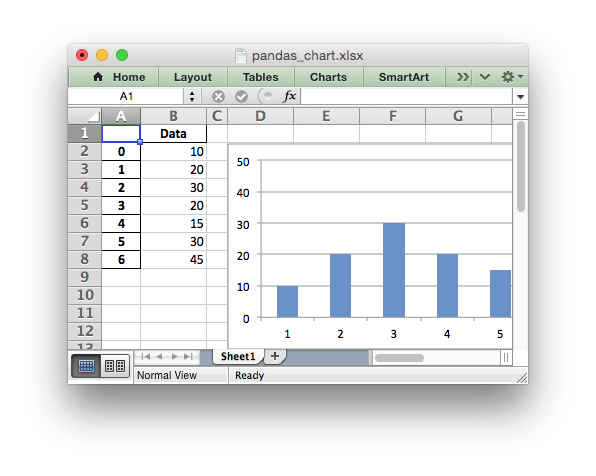# Example: Pandas Excel output with a chart

A simple example of converting a Pandas dataframe to an Excel file with a chart using Pandas and XlsxWriter.```##############################################################################
#
# An example of converting a Pandas dataframe to an xlsx file with a chart
# using Pandas and XlsxWriter.
#
# Copyright 2013-2023, John McNamara, jmcnamara@cpan.org
#

import pandas as pd

# Create a Pandas dataframe from some data.
df = pd.DataFrame({"Data": [10, 20, 30, 20, 15, 30, 45]})

# Create a Pandas Excel writer using XlsxWriter as the engine.
writer = pd.ExcelWriter("pandas_chart.xlsx", engine="xlsxwriter")

# Convert the dataframe to an XlsxWriter Excel object.
df.to_excel(writer, sheet_name="Sheet1")

# Get the xlsxwriter workbook and worksheet objects.
workbook = writer.book
worksheet = writer.sheets["Sheet1"]

# Create a chart object.